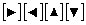##### TI-84 Plus CE Graphing Calculator For Dummies, 3rd EditionFollowing is a list of ten common error messages the TI-84 Plus calculator may give you. When you get an error, pay close attention to the error message screen. Your calculator displays a descriptive note on the error screen that helps you determine the cause of the error.

• ARGUMENT

You usually get this message when you are using a function housed in one of the menus on the calculator. This message indicates that you have not properly defined the argument needed to use the function.

To avoid this error, take advantage of the Catalog Help feature. Use thekeys to navigate your cursor to the function you want and press [+] to view the syntax of the function you want.

This message indicates that the guess you’ve given to the calculator isn’t within the range of numbers that you specified. This is one of those times when the calculator asks you to guess the solution. One example is when you’re finding the maximum value or the zero of a function within a specified range. Another is when you’re finding the solution to an equation where that solution is contained in a specified range.

One other time that you can get this message is when the function is undefined at (or near) the value of your guess.

• DIMENSION MISMATCH

You usually get this message when you attempt to add, subtract, or multiply matrices that don’t have compatible dimensions.

You also get this error if you try to graph a scatter plot of data lists that don’t have the same dimensions. In other words, the number of elements in L1 and L2 are not the same.

• DOMAIN

You usually get this message when you’re using a function housed on a menu of the calculator. If that function is, for example, expecting you to enter a number in a specified range, you get this error message if that number isn’t in the specified range.

When using the Finance app, you get this message when you don’t use the correct sign for cash flow.

• INVALID

This is the catchall error message. Basically, it means that you did something wrong when defining something (for example, you used function Y3 in the definition of function Y2, but forgot to define function Y3).

• INVALID DIMENSION

You get this invalid-dimension message if (for example) you attempt to raise a nonsquare matrix to a power or enter a decimal for an argument of a function when the calculator is expecting an integer.

You can also get this error if you inadvertently leave the stat plots on when you are trying to graph a function. Turn the stat plots off by pressing [2nd][Y=].

• NO SIGN CHANGE

When you’re using the Equation Solver, you get this message when the equation has no real solutions in your specified range.

• SINGULAR MATRIX

You get this message when you try to find the inverse of a matrix whose determinant is zero.

• SYNTAX

This is another catchall error message. It usually means you have a typo somewhere or you have done something the calculator wasn’t expecting.

• WINDOW RANGE

This, of course, means that the Window is improperly set. This problem is usually (but not always) caused by improperly setting Xmin greater than or equal to Xmax or Ymin greater than or equal to Ymax in the Window editor.